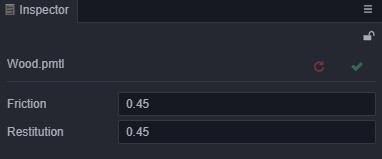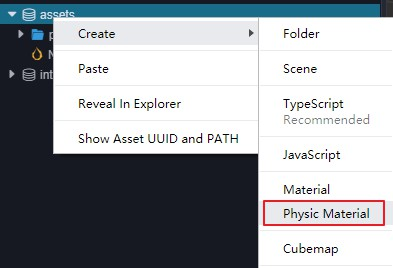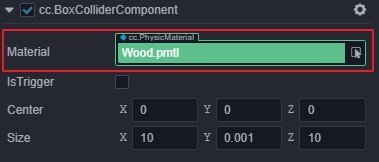# Physics Material

In Cocos Creator, the physics material is a asset, which records the surface information of the object. This is used to calculate the friction and elastic force of the colliding object.

## Properties of Materials

The properties of Physics Materials are shown below:Properties Description
friction Coefficient of friction
restitution Coefficient of restitution

When in contact with other surfaces, these coefficients are used to calculate the corresponding friction and elastic forces.

## Creating Physics Materials

Physics Materials can be created in two ways:

1. Create in editor
2. Code instantiation

The way to create with the editor is shown below:Instantiated in the code:

``````let newPmtl = new PhysicMaterial();
newPmtl.friction = 0.1;
newPmtl.restitution = 0.1;
``````

## Application of materials

The physics material is set in units of collision bodies, and each `Collider` has a `material` property (when not set, `Collider` will refer to the default physics material in the physics system). The application to `Collider` is also divided into editor operation and code operation.

To operate in the editor, just drag the asset into the `cc.PhysicsMaterial` property box, as shown in the following figure:Operation in the code:

``````const collider = this.node.getComponent(ColliderComponent);
collider.material = newPmtl;
``````

Because of the design of Material Sharing, you can actually do this directly in the code (because an instance will be created when you get `material`)

``````collider.material.friction = 0.1;
collider.material.restitution = 0.1;
``````